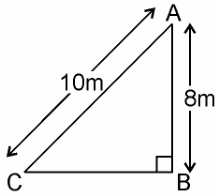# A ladder 10 m long reaches a window 8 m above the ground. Find the distance of the foot of the ladder from base of the wall.

Given

A ladder 10 m long reaches a window 8 m above the ground.

Find out

The distance of the foot of the ladder from base of the wall.

SolutionAB is wall = 8m

BC = ?

In right ∆ABC, by Pythagoras theorem

(AC)= (AB)+ (BC)2

(10)= (8)+ (BC)2

100 = 64 + (BC)2

100 – 64 = (BC)2

36 = (BC)2

√36 = BC

√(6 * 6) = BC

6 = BC

Hence, the foot of the ladder is at a distance of 6 m from the base of the wall.(0)(0)